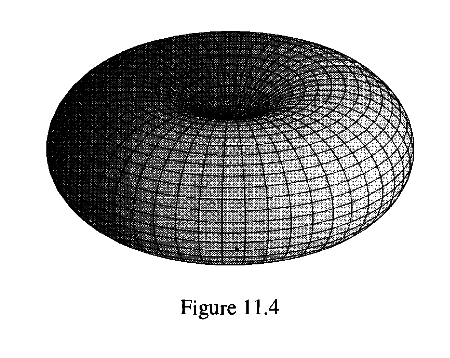Brian Bi

## Generally speaking, what determines a photons vector? Why does it go whizzing off at the speed of light in one direction vs. another?

What determines a photon's vector? Why does [a photon] go whizzing off at the speed of light in one direction vs. another?

The laws of electromagnetism determine it... in probability. Photons are produced and consumed in electromagnetic interactions. A theory of electromagnetism must provide the principles necessary to answer this question.

It is easier to start with the classical picture first. When an electromagnetic wave has a definite wave vector $k$, it has electric and magnetic fields that are mutually perpendicular to each other and to the wave vector. Conversely, the direction of energy flow in an electromagnetic wave is perpendicular to both the electric and magnetic fields.

A simple case we can reason out is that of a charged particle oscillating back and forth. Say the particle is located near the origin and is oscillating in the z direction. If the particle is oscillating in the z direction, then the electric field on the z axis must also be in the z direction. If it pointed in any other direction, it would violate rotational symmetry. Now, since the direction of energy flow must be perpendicular to the z direction, there can be no flow of energy along the z axis. So there is no radiation in the direction of oscillation.

A diagram in Griffiths illustrates this. The radiation emitted has the greatest intensity perpendicular to the dipole moment. The intensity goes to zero near the z axis, parallel to the dipole moment.In general, Maxwell's equations determine the electric and magnetic fields around a radiation source, and the magnitude and direction of the electric and magnetic fields encode the information about the rate and direction in which electromagnetic energy is flowing.

When electromagnetism is quantized, we end up with similar results. Namely, the oscillating particle emits photons in various directions, but the distribution has to look like the classical one when the photon number is large. That is, the greatest number of photons will be emitted perpendicular to the dipole moment, and no photons at all will be emitted along the axis. Each photon travels in a random, uncertain direction but is most likely to be nearly perpendicular to the axis. The direction becomes certain when the photon is observed. In general, the theory of quantum electrodynamics can be used to calculate properties of the photon field $A$ including the probability of finding $N$ photons of any given wave vector $k$ and polarization $\epsilon$, although these calculations are very difficult to do exactly.